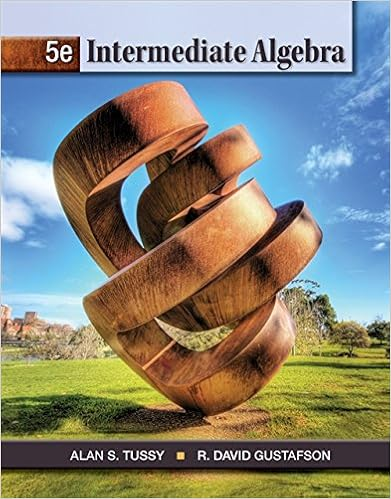# 1159 pm mountain time on monday evening intellectual

• Notes
• 17

This preview shows page 7 - 11 out of 17 pages.

##### We have textbook solutions for you!
The document you are viewing contains questions related to this textbook.The document you are viewing contains questions related to this textbook.
Chapter 6 / Exercise 34
Intermediate Algebra
Tussy/GustafsonExpert Verified
11:59 PM Mountain Time on Monday evening Intellectual Elaboration: Hello Class: Welcome to Week 1! Let’s start by talking about rational expressions. A rational expression is any expression that can be written as a quotient of two polynomials. Here are some examples: Rational expressions are examples of algebraic fractions. They are also examples of fractional expressions. Because rational expressions indicate division, we must be careful to avoid denominators that are 0. Proprietary Information of Bridgepoint Education, Inc. Created by Learning and Development – Academics NL-3.23.2012 7
##### We have textbook solutions for you!
The document you are viewing contains questions related to this textbook.The document you are viewing contains questions related to this textbook.
Chapter 6 / Exercise 34
Intermediate Algebra
Tussy/GustafsonExpert Verified
Let’s consider this example Therefore the expression is undefined at x= 7 and x = -4 Example 1 (Bittinger & Ellenbogen, 2006) A rational expression is said to be simplified when the numerator and the denominator have no factors (other than 1) in common. To simplify a rational expression, we first factor the numerator and denominator. We then identify factors common to the numerator and denominator, rewrite the expression as a product of two rational expressions (one of which is equal to 1), and then remove the factor equal to 1. Canceling is a shortcut that can be used—and easily misused —to simplify rational expressions. Canceling must be done with care and understanding. Essentially, canceling streamlines the process of removing a factor equal to 1. Working with rational expressions is exactly the same as working with fractions. All the same rules are in play. The Product of Two Rational Expressions Proprietary Information of Bridgepoint Education, Inc. Created by Learning and Development – Academics NL-3.23.2012 8
To multiply rational expressions, multiply numerators and multiply denominators: The Quotient of Two Rational Expressions To divide by a rational expression, multiply by its reciprocal: The Sum of Two Rational Expressions: To add when the denominators are the same, add the numerators and keep the common denominator: The Difference of Two Rational Expressions: To subtract when the denominators are the same, subtract the second numerator from the first and keep the common denominator: Proprietary Information of Bridgepoint Education, Inc. Created by Learning and Development – Academics NL-3.23.2012 9 x x
Least Common Multiples and Denominators: To add or subtract rational expressions that have different denominators, we must first find equivalent rational expressions that do have a common denominator. The least common multiple must include the factors of each number, so it must include each prime factor the greatest number of times that it appears in any factorizations. To Find the Least Common Denominator (LCD) 1. Write the prime factorization of each denominator. 2. Select one of the factorizations and inspect it to see if it contains the other.
•••